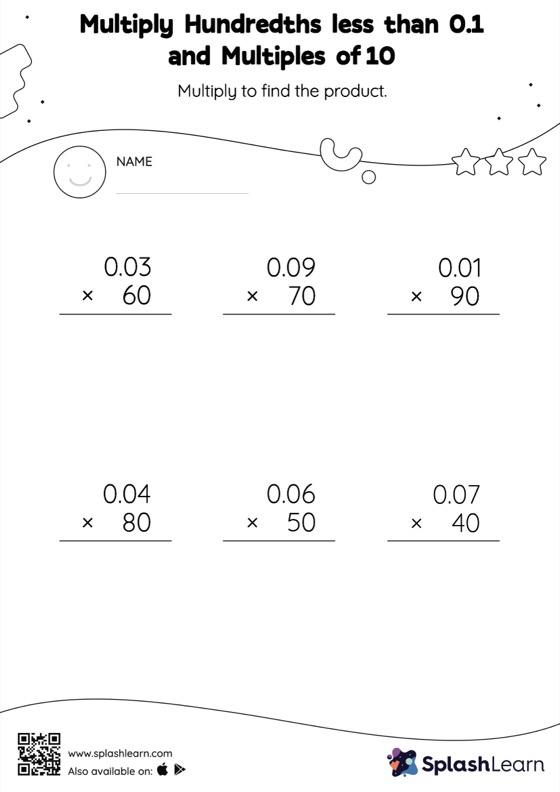# Multiply Hundredths less than 0.1 and Multiples of 10: Vertical Multiplication Worksheet

Home > Multiply Hundredths less than 0.1 and Multiples of 10: Vertical MultiplicationHelp your little one develop a knack for math with this multiply hundredths less than 0.1 And multiples of 10 worksheet. Students know that when multiplying a decimal by a number ending in zeros, the decimal point is first ignored and then placed in the product as many places from the right as the number of decimal digits in the original number. They practice this idea thoroughly in multiply hundredths less than 0.1 And multiples of 10 worksheet. This worksheet is about practicing the column method in which numbers are written one on top of another. In this method, students use their place value understanding to solve the problems.